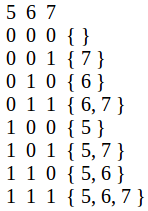Related Articles

# Find sum of sum of all sub-sequences

• Difficulty Level : Easy
• Last Updated : 09 Jun, 2021

Given an array of n integers. The task is to find the sum of each sub-sequence of the array.
Examples :

```Input : arr[] = { 6, 8, 5 }
Output : 76
All subsequence sum are:
{ 6 }, sum = 6
{ 8 }, sum = 8
{ 5 }, sum = 5
{ 6, 8 }, sum = 14
{ 6, 5 }, sum = 11
{ 8, 5 }, sum = 13
{ 6, 8, 5 }, sum = 19
Total sum = 76.

Input  : arr[] = {1, 2}
Output : 6```

Method 1 (brute force):
Generate all the sub-sequence and find the sum of each sub-sequence.
Method 2 (efficient approach):
For an array of size n, we have 2^n sub-sequences (including empty) in total. Observe, in total 2n sub-sequences, each element occurs 2n-1 times.
For example, arr[] = { 5, 6, 7 }So, the sum of all sub-sequence will be (sum of all the elements) * 2n-1.
Below is the implementation of this approach:

## C++

 `// C++ program to find sum of all sub-sequences``// of an array.``#include``using` `namespace` `std;` `// Return sum of sum of all sub-sequence.``int` `sum(``int` `arr[], ``int` `n)``{``  ``int` `ans = 0;` `  ``// Finding sum of the array.``  ``for` `(``int` `i = 0; i < n; i++)``    ``ans += arr[i];` `  ``return` `ans * ``pow``(2, n - 1);``}` `// Driver Code``int` `main()``{``  ``int` `arr[] = { 6, 7, 8 };``  ``int` `n = ``sizeof``(arr)/``sizeof``(arr);` `  ``cout << sum(arr, n) << endl;` `  ``return` `0;``}`

## Java

 `// Java program to find sum of``// all sub-sequences of an array.``import` `java.io.*;``import` `java.math.*;` `class` `GFG {``    ` `    ``// Return sum of sum of all sub-sequence.``    ``static` `int` `sum(``int` `arr[], ``int` `n)``    ``{``    ``int` `ans = ``0``;``    ` `    ``// Finding sum of the array.``    ``for` `(``int` `i = ``0``; i < n; i++)``        ``ans += arr[i];``    ` `    ``return` `ans * (``int``)(Math.pow(``2``, n - ``1``));``    ``}``    ` `    ``// Driver Code``    ``public` `static` `void` `main(String args[])``    ``{``    ``int` `arr[]= { ``6``, ``7``, ``8` `};``    ``int` `n = arr.length;``    ` `    ``System.out.println(sum(arr, n));``    ``}``}``    ` `// This code is contributed by Nikita Tiwari.`

## Python3

 `# Python 3 program to find sum of``# all sub-sequences of an array.`  `# Return sum of sum of all sub-sequence.``def` `sm(arr , n) :``    ``ans ``=` `0` `    ``# Finding sum of the array.``    ``for` `i ``in` `range``(``0``, n) :``        ``ans ``=` `ans ``+` `arr[i]``    ` `    ``return` `ans ``*` `pow``(``2``, n ``-` `1``)``    ` `    ` `# Driver Code``arr ``=` `[ ``6``, ``7``, ``8` `]``n``=``len``(arr)` `print``(sm(arr, n))`  `# This code is contributed by Nikita Tiwari.`

## C#

 `// C# program to find sum of``// all sub-sequences of an array.``using` `System;` `class` `GFG``{``    ` `    ``// Return sum of sum of all sub-sequence.``    ``static` `int` `sum(``int` `[]arr, ``int` `n)``    ``{``    ``int` `ans = 0;``    ` `    ``// Finding sum of the array.``    ``for` `(``int` `i = 0; i < n; i++)``        ``ans += arr[i];``    ` `    ``return` `ans * (``int``)(Math.Pow(2, n - 1));``    ``}``    ` `    ``// Driver Code``    ``public` `static` `void` `Main()``    ``{``    ``int` `[]arr= { 6, 7, 8 };``    ``int` `n = arr.Length;``    ` `    ``Console.Write(sum(arr, n));``    ``}``}``    ` `// This code is contributed by nitin mittal`

## PHP

 ``

## Javascript

 ``

Output:

`84`

This article is contributed by Anuj Chauhan. If you like GeeksforGeeks and would like to contribute, you can also write an article using write.geeksforgeeks.org or mail your article to review-team@geeksforgeeks.org. See your article appearing on the GeeksforGeeks main page and help other Geeks.